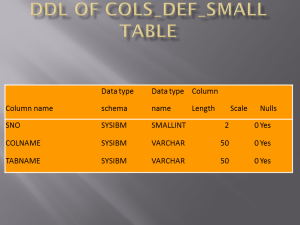# When I had to write a procedure for simple ‘ALTER COLUMN’ statements in DB2

Problem : Changing datatype of more than one column for one table and not running reorg after every alter column statement as the table is having millions of records.
Scenario : I have been busy recently in migrating data from AS400 to DB2 UDB 10.5 LUW. I mapped all the columns which were numeric and decimal type having 0 precision such as (1,0) or (2,0) to smallint if there length was between 1 to 5. If the length were between 5 and 10, I mapped them to integer . The length of numeric and decimal type having length more 10 , I mapped them to BIGINT. But here comes a problem the developer has defined all the columns as java type INT . So other then 5 or 6 columns I had to change all the SMALLINT columns to INTEGER type .
Solution : I took all the columns which were smallint type by executing this SQL:
SELECT tabschema, tabname, colname FROM syscat.columns where TYPENAME = ‘SMALLINT’ AND colname NOT IN (‘col1′,’col2’) order by tabname, colname
Once I got the data , from above SQL in a table using IMPORT statement , I wrote a small stored procedure by name of CRT_ALTER_STMT_FROM_SMALL_TO_INT . Attached is the code :
COLS_DEF_SMALL is the table which is used in the STORED PROCEDURE , where columns having SMALLINT datatype are loaded. DDL of COLS_DEF_SMALL table is as :CREATE or REPLACE PROCEDURE CRT_ALTER_STMT_FROM_SMALL_TO_INT()
RESULT SETS 1
Language SQL
SPECIFIC CRT_ALTER_STMT_FROM_SMALL_TO_INT
BEGIN
DECLARE v_tabname varchar(100) default ‘ ‘;
DECLARE v_colname varchar(100) default ‘ ‘;
DECLARE v_max_ctr BIGINT default 0;
DECLARE v_min_ctr BIGINT default 0;
DECLARE v_sno BIGINT default 0;
DECLARE v_ctr BIGINT default 1;
DECLARE v_desc varchar(300) default ‘ ‘;
DECLARE v_col_desc varchar(300) default ‘ ‘;

DECLARE result_ddl cursor with return for select desc
from DB2ADMIN.new_generated_ddl order by seq_no with ur;

For re as select tabname, colname, sno from cols_def_small
order by tabname,sno DO
set v_tabname = tabname;
set v_colname = colname;
set v_sno = sno;

select min(sno), max(sno) into v_min_ctr , v_max_ctr
from cols_def_small where tabname = v_tabname;

If v_sno = v_min_ctr then
set v_desc = ‘ALTER TABLE FMCLIB.’||v_tabname;
set v_ctr = v_ctr+1;
Insert into DB2ADMIN.new_generated_ddl (desc,seq_no) values (RTRIM(v_desc), (v_ctr+1));
END IF;

If v_sno <> v_max_ctr then
set v_col_desc = ‘ALTER COLUMN ‘|| v_colname || ‘ SET DATA TYPE INTEGER’;
set v_ctr = v_ctr+1;
Insert into DB2ADMIN.new_generated_ddl (desc,seq_no) values (RTRIM(v_col_desc), (v_ctr+1));
END IF;

IF v_sno = v_max_ctr then
set v_col_desc = ‘ALTER COLUMN ‘|| v_colname || ‘ SET DATA TYPE INTEGER’;
set v_ctr = v_ctr+1;
Insert into DB2ADMIN.new_generated_ddl (desc,seq_no) values (RTRIM(v_col_desc), (v_ctr+1));
set v_col_desc= ‘;’;
set v_ctr = v_ctr+1;
Insert into DB2ADMIN.new_generated_ddl (desc,seq_no) values (RTRIM(v_col_desc),(v_ctr+1));
set v_ctr = v_ctr+1;
set v_col_desc= ‘REORG TABLE FMCLIB.’|| v_tabname ||’;’;
Insert into DB2ADMIN.new_generated_ddl (desc,seq_no) values (RTRIM(v_col_desc), (v_ctr+1));
set v_desc = ‘ ‘;
set v_col_desc=’ ‘;
set v_tabname = ‘ ‘;
End IF;
END FOR;
open result_ddl ;
END@
Advantage : For all the tables and for all the columns type I was able to create one script, in which I could alter the column types as well as run the REORG and RUNSTATS.